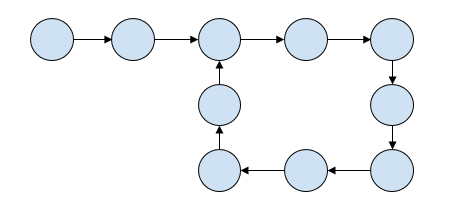# Find length of loop in linked list in C++

In this problem, we are given a linked list that might contain loops. Our task is to find the length of the loop in the linked list.

Problem Description: we need to count the number of nodes in the loop if it contains a loop otherwise return -1.

Let’s take an example to understand the problem,Output: 8

## Solution Approach

To solve the problem, we first need to check whether the linked list contains a loop. An approach to check this is using Floyd’s Cycle Finding Algorithm.

In Floyd’s Cycle Finding Algorithm, we will traverse the linked list using two pointers. One slowPointer that increases by 1 and another one is fastPointer that increases by 2. If both numbers meet at some point then there exists a loop otherwise not.

If there exists a loop, we need to count the number of nodes that are present in the loop. For this we will start from the point where slowPointer and fastPointer meet and then count the number of nodes traversed to get to the position back.

## Example

Live Demo

#include<bits/stdc++.h>
using namespace std;

struct Node {
int data;
struct Node* next;
};

int countLoopNodespoint(struct Node *n) {
int res = 1;
struct Node *temp = n;
while (temp->next != n) {

res++;
temp = temp->next;
}
return res;
}

int countLoopNode(struct Node *list) {

struct Node *slowPtr = list, *fastPtr = list;
while (slowPtr && fastPtr && fastPtr->next) {
slowPtr = slowPtr->next;
fastPtr = fastPtr->next->next;

if (slowPtr == fastPtr)
return countLoopNodespoint(slowPtr);
}
return 0;
}

struct Node *newNode(int key) {
struct Node *temp = (struct Node*)malloc(sizeof(struct Node));
temp->data = key;
temp->next = NULL;
return temp;
}

int main() {
}
The number of nodes in the loop are 6>]